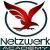true

Learn Data Science from the Best Tutors

•Affordable fees
•1-1 or Group class
•Flexible Timings
•Verified Tutors

Search in

# Mathematics used in various Machine learning conceptsAkash L kulkarni
19/05/2020 00

Mathematics is the building block for data science. This blog focuses on various mathematical concepts that are used in machine learning. The mathematical concepts used for machine learning are categorized into statistics, probability, differential calculus. Let’s discuss one by one.

## 1.Statistics

In mathematical terms, statistics is defined as the set of equations, which are helpful to interpret and analyze things.  In machine learning, statistics plays a very important role in understanding the data in a dataset. Various statistical analysis helps us to understand the distribution, summary, etc. of data.

### 1.1.Exploratory data analysis

EDA or exploratory data analysis is one of the critical steps in data science. It helps us to analyze the data patterns, errors, outliers, etc. Statistics being the backbone for this step, various concepts such as standard deviation, variance, mean, median, etc. are used.

We consider data that is outside three standard deviations (In general) as the outliers. We understand data distribution by plotting a bar graph, which helps us understand whether data is distributed across mean or is the data skewed towards one side.

## 2.Probability

Probability is the branch of mathematics which is concerned with the numerical description of explaining how likely an event is to occur. This theory is very useful in making predictions. Estimation and predictions constitute an important part of Data Science, and thus, most of the concepts involve probability theory.

## 2.1.Classification algorithms

Most of the classification problems in data science involve the predictions of classes, where we classify each observation to exactly one class. The base idea behind the classification problem is probability. The probabilities of all the classes are calculated based on the trained data; the class with the highest probability is assigned to that observation.

## 2.2.Loss function

One of the loss functions used for classification problems is the cross-entropy loss which is a measure of the classification model. Cross-entropy loss increases as the predicted probability diverge from the actual label. It is one of the most important calculations when it comes to machine learning for classification.

## 3.Differential calculus

Data science is incomplete without differential calculus. Differentiation forms an intrinsic part of data science, especially in machine learning. Differentiation or calculus is the study of the rate of changes in quantities.

In machine learning, our goal is to reduce the cost to our input data. We use cost function, which is the measure of the error in the predictions of the model. To achieve the lowest possible value of the cost function is the main goal of gradient descent which in turn improves the accuracy. Gradient descent uses differentiation where the partial derivative of the cost function is calculated, which will point to the global minima. The downfall of the gradient is controlled by the learning rate.

The same concept is applied for deep learning models where the optimizer used as gradient descent will use the partial derivative concept to adjust the weights to get the optimal weights.

0 Dislike
Follow 2## Other Lessons for You

4 Key Things to Learn for Data Science
1. Theory:Use Coursera and EdX for theory, concepts, and applications of probability, statistics, linear algebra, calculus, and machine learning.2. Data Visualisation:Tableau and PowerBI are easy-to-use...TOP 10 Tools for Data Science
TOP 10 Tools for Data Science1. Python2. SQL3. R4. Tableau5. PowerBI6. Java7. Julia8. Scala9. SAS10. ExcelTOP 10 Websites for Data Science1. Coursera3. EdX4. Udacity5. Kaggle6. Analytics Vidhya7. KDNuggets8....What is Time Series?
What is a Time Series? Time Series data is a series of data points indexed or listed or graphed with an equally spaced period. Time series forecasting is the use of the model to predict future values...Outlier
Outliers* An Outlier is an observation point that is distant from other observations.* An outlier may indicate an experimental error, or it may be due to variability in the measurement. * Outliers are...Regularisation in Machine Learning
Regularization In Machine Learning, Regularization is the concept of shrinking or regularizing the coefficients towards zero. It helps the model to prevent overfitting. Overfitting in Machine Learning...### Looking for Data Science Classes?

Learn from Best Tutors on UrbanPro.

Are you a Tutor or Training Institute?

Join UrbanPro Today to find students near you
X

### Looking for Data Science Classes?

The best tutors for Data Science Classes are on UrbanPro

• Select the best Tutor
• Book & Attend a Free Demo
• Pay and start Learning### Learn Data Science with the Best Tutors

The best Tutors for Data Science Classes are on UrbanPro